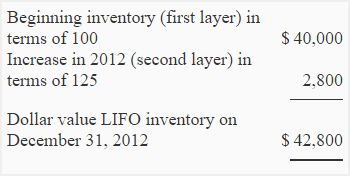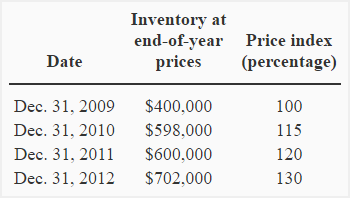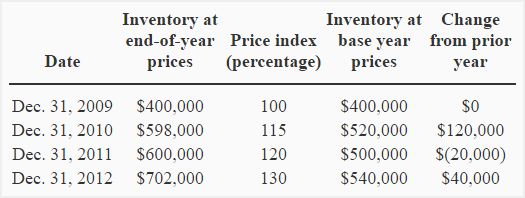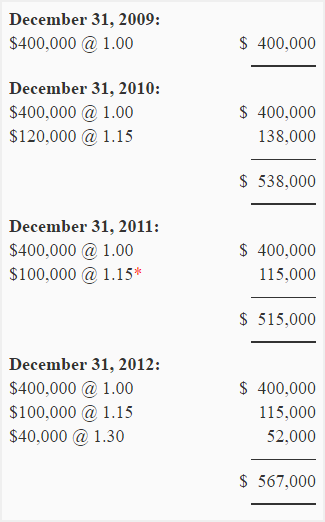# Dollar-value LIFO method

Like specific goods pooled LIFO approach, Dollar-value LIFO method is also used to alleviate the problems of LIFO liquidation. Under this method, goods are combined into pools and all increases and decreases in a pool are measured in terms of total dollar value. The pools created under this method are, therefore, known as dollar-value LIFO pools.

This approach is considered more effective than the specific goods pooled LIFO approach (discussed in ‘LIFO liquidation’ article) because of the following reasons:

1. As the pools are determined and measured in terms of total dollar value, this method allows companies to include a broader range of goods in a pool.
2. Under specific goods pooled LIFO approach, an item can be replaced only with an item that is substantially identical whereas in a dollar-value LIFO pool, an item can be replaced with an item that is similar in use or interchangeable.

Under this method, it is possible to use a single pool. However, companies can use any number of pools according to their requirement. The unnecessary employment of a large number of dollar-value LIFO pools  may increase the cost and also reduce the effectiveness of dollar-value LIFO approach.

## Who uses dollar-value LIFO method?

The companies that maintain a large number of products and expect significant changes in their product mix in future, frequently use dollar-value LIFO technique. The use of traditional LIFO approaches is common among companies that have a few items and expect very little change in their product mix.

Consider the following example to understand how the value of inventory is computed under dollar value LIFO method:

## Example 1:

The Fast company adopted dollar-value LIFO method on December 31, 2011. The inventory on current prices at the end of 2011 and 2012 was as follows:

December 31, 2011(end of year prices): \$40,000

December 31, 2012 (end of year prices): \$52,800

The inventory prices were increased by 25% during the year 2012.

Required: Compute the amount of inventory at the end of 2012 using dollar-value LIFO method.

### Solution:

First of all, we need to compute the value of ending inventory at base-year-prices. It is computed using the following formula:

Ending inventory at base-year-prices = \$52,800/1.25

= \$42,240

Now we can compute the real-dollar quantity increase in inventory:

= (\$42,240 – \$40,000)

= \$2,240

The next step is to value this real dollar quantity increase in inventory at year-end-prices:

= \$2,240 × 1.25

= \$2,800

The real dollar quantity increase in inventory valued at year-end-prices is usually known as dollar-value LIFO layer (or layer). If this layer is added to the beginning inventory of the year 2012,  we would get the total inventory at the end of the  year 2012. It is shown below:Hint!
A layer is formed only when ending inventory at base-year-prices exceeds the beginning inventory at base-year-prices.

## Example 2 – the use of dollar-value LIFO method in a more complex situation:

The following information belongs to Best Buy company:Required: Compute the inventory at the end of each year using dollar-value LIFO method.

### Solution:

First, we need to add two new columns to the original information given in the  example; one to show the inventory at base-year-prices and one to show the changes in inventory from prior year. It is done as follows:Now we can compute the dollar value inventory:* The price index of 2010 has been used because no layer has been formed during the year 2011. The ending inventory at base-year-prices (\$500,000) is less than the beginning inventory at base-year-prices (\$520,000).

Show your love for us by sharing our contents.

### 11 Comments on Dollar-value LIFO method

1.Fatoom

This was very helpful. Thanks a bunch!

2.Dave

Agreed. Very helpful! 🙂

3.Bhushan

Thanks for the detailed explanation…

4.Ahi

This’s got a lot of clarity, thanks a lot.

5.leah

The best explanation! Thank you very much!

6.Martha

Finally got this with your explanation and example. Previously had a difficult time understanding this from both the textbook and the professor’s explanations. Thank you!

7.mao

thank you so much for such detailed explanation. have been having problems in understanding this topic when studying for CPA. totally forget it what had learnt in school.

8.Val

Should the last one be:
December 31, 2012:
\$400,000 @ 1.00 = \$400,000
\$120,000 @ 1.15 = \$138,000*
\$20,000 @ 1.30 = \$26,000*
————-
\$564,000*
————-

*corrected

9.Abbas

Great Article. Thanks for detailed explanation.

10.will

Do you have to report LIFO liquidation profits on financial statements
when using Dollar Value LIFO?

11.Marlene Flax

Thank you so much for clear examples of Dollar Value LIFO and its explanations.